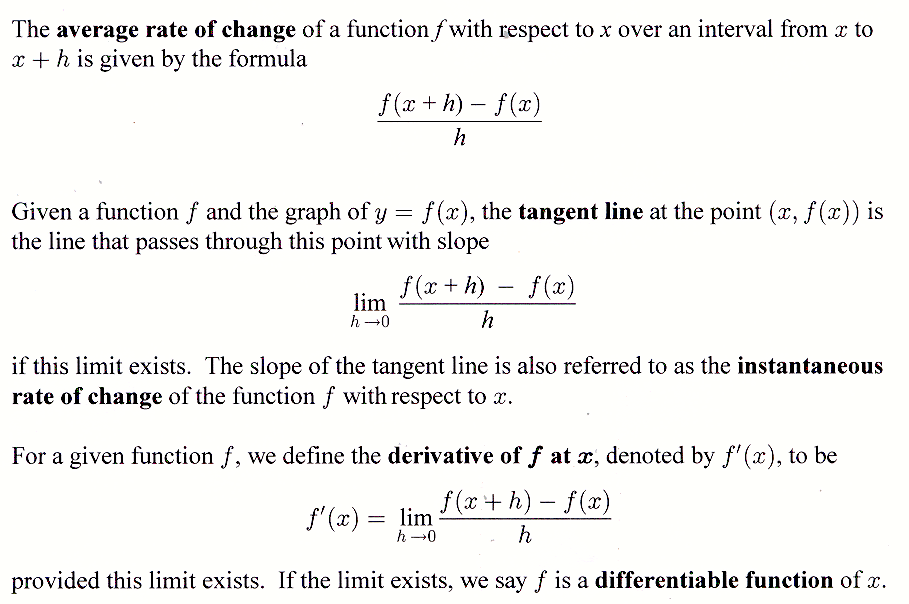# Section 18.3: Derivatives

## 18.3 Outline

1. Average rate of change
2. Instantaneous rate of change
3. Derivative
1. derivative of f at x
2. differentiable function
3. derivative of ex
4. Tangent line
1. tangent-line
formula
2. equation of tangent line at a point (h, k ).
5. Velocity

## 18.3 Essential Ideas